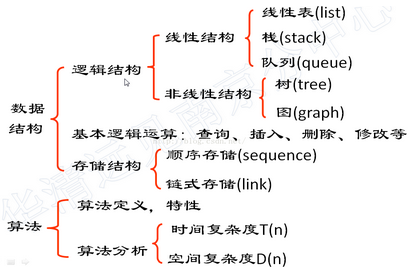• 欢迎访问BruceOu的博客，推荐使用最新版火狐浏览器和Chrome浏览器访问本网站
• 如果您觉得本站非常有看点，那么赶紧使用Ctrl+D 收藏本章吧

# 《数据结构与算法》第1章 数据结构与算法分析概述(C语言)

2周前 (06-04) 17次浏览## 1.1数据结构概述

### 1.1.2数据结构的基本术语

1. 数据（Data）

2. 数据元素（Data Element）

3. 数据对象（Data Object）

4. 数据结构（Data Structure）

5. 数据的逻辑结构（Logical Structure）

6. 数据的存储结构（Physical Structure）

7. 数据类型（Data Type）

8. 抽象数据类型（Abstract Data Type）

## 1.2算法分析概述### 1.2.1算法的特性

1）有穷性 ——算法执行的步骤（或规则）是有限的；
2）确定性 ——每个计算步骤无二义性；
3）可行性——每个计算步骤嫩巩固在有限的时间内完成；
4）输入——算法有一个或多个外部输入；
5）输出——算法有一个或多个输出；

### 1.2.2评价一个算法的好坏

1）消耗时间的多少；
2）消耗存储空间的多少；
3）算法的设计是否容易理解，是否容易编程实现，方便调试和维护；

### 1.2.3时间复杂度

1）问题的规模：输入数据量的大小，用n来表示；
2）算法的时间复杂度：算法消耗时间，它是问题规模的函数 T （n）。

#### 1.2.3.1语句的频度

void MATRIXM(A,B,C)
{
float A[n][n],B[n][n],C[n][n];
int i,j,k;                                                 // 语句频度
for(i = 0;i < n; i++)                                      //   n+1
for(j = 0;j < n;j++)                               //  n(n+1)
{
C[i][j] = 0;                                  //   n*n
for(k = 0; k < n;k++)                        //  n*n(n+1)
C[i][j] = c[i][j]+A[i][k]*B[k][j];     //   n*n*n
}
}


#### 1.2.3.2算法的时间复杂度

T(n) = (n+1)+n(n+1) + n *n+n * n(n+1)+n * n * n

= 2 * n * n * n + 3 * n * n +2 * n +1

T(n) = O (f(n))

BrueceOu , 版权所有丨如未注明 , 均为原创丨本网站采用BY-NC-SA协议进行授权

[支付宝]• 版权声明

本站的文章和资源来自互联网或者站长
的原创，按照 CC BY -NC -SA 3.0 CN
协议发布和共享，转载或引用本站文章
应遵循相同协议。如果有侵犯版权的资
源请尽快联系站长，我们会在24h内删
除有争议的资源。
• 网站驱动

• 友情链接

• 支持主题